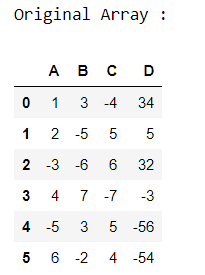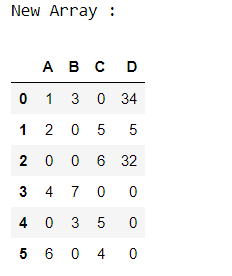# Replace Negative Number by Zeros in Pandas DataFrame

In this article, Let’s discuss how to replace the negative numbers by zero in Pandas

Approach:

• Import pandas module.
• Create a Dataframe.
• Check the DataFrame element is less than zero, if yes then assign zero in this element.
• Display the final DataFrame

First, let’s create the dataframe.

## Python3

 `# importing pandas module ` `import` `pandas as pd ` ` `  `# Creating pandas DataFrame ` `df ``=` `pd.DataFrame({``"A"``: [``1``, ``2``, ``-``3``, ``4``, ``-``5``, ``6``], ` `                   ``"B"``: [``3``, ``-``5``, ``-``6``, ``7``, ``3``, ``-``2``], ` `                   ``"C"``: [``-``4``, ``5``, ``6``, ``-``7``, ``5``, ``4``], ` `                   ``"D"``: [``34``, ``5``, ``32``, ``-``3``, ``-``56``, ``-``54``]}) ` ` `  `# Displaying the original DataFrame ` `print``(``"Original Array : "``) ` `df `

Output :Now, let’s find the negative element and replace it with zero.

## Python3

 `# checking the element is < 0 ` `df[df < ``0``] ``=` `0` `print``(``"New Array :"``) ` `df `

Output :Whether you're preparing for your first job interview or aiming to upskill in this ever-evolving tech landscape, GeeksforGeeks Courses are your key to success. We provide top-quality content at affordable prices, all geared towards accelerating your growth in a time-bound manner. Join the millions we've already empowered, and we're here to do the same for you. Don't miss out - check it out now!

Previous
Next